Purva Huilgol — Updated On January 6th, 2022

## Overview

• Data manipulation is one of the most crucial steps one has to perform in the machine learning lifecycle
• Let’s learn to use  the most widely-used set of apply functions for transforming data in R

## Introduction

Data manipulation is one of the most crucial steps one has to perform in the machine learning lifecycle. It entails transforming the provided data so that it can be used for building predictive models.

Additionally, it is here that a skilled data scientist applies their intuition and experience to extract as much information from the data as possible. Thus, it should come as no surprise that there exists a plethora of functions and tools in both Python and R to help us in this task.Featured Image

Today, we will be using R and learning about the most widely-used set of ‘apply‘ functions for transforming data in R. This family of functions offers efficient and quick operations on data. This is particularly useful when we want to work only with certain columns. This set of functions is called the apply() functions. Along with its variants like sapply(), mapply(), etc., we are provided a multipurpose swiss-army knife for data manipulation.

If you are interested in having a career in Data Science and learning about these amazing things, I recommend you check out our Certified AI & ML BlackBelt Accelerate Program.

The various set of functions in this family are:

• Setting the Context
• apply
• lapply
• sapply
• vapply
• tapply
• mapply

## Setting the Context

I will first cover how each function above works by using simple datasets and then we will take up a real dataset to use these functions.

So fire up your Notebooks or R studio, and let us get started!

We don’t need to install any other libraries to use the apply functions. So let us start by creating a simple matrix of numerical values from 1 to 20 distributed among 5 rows and 4 columns:

```data <- matrix(c(1:20), nrow = 5 , ncol = 4)
data```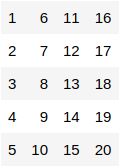This is how our matrix looks. Now, let us start with the apply() function

## apply()

The general syntax of the apply() function can be obtained using the help section. Just execute this code to get a detailed documentation

`?apply`

As we can see, the apply function has the structure of apply(X, MARGIN, FUN, …). Here,

• X refers to the dataset(in our case the matrix) we will be applying the operations on
• MARGIN parameters allow us to specify if we want to apply the operation by the row or by the column.
• MARGIN = 1 for rows
• MARGIN = 2 for columns
• FUN refers to any user-defined or in-built function we want to ‘apply’ on X

Let us look at the simple example of calculating the mean of each row:

```mean_rows <- apply(data, 1, mean)
mean_rows```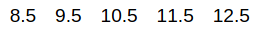That was fairly simple! We can see how the apply() function can be used to summarise our data. In the same vein, Let us try finding e sum along each column:

```sum_cols <- apply(data, 2, sum)
sum_cols```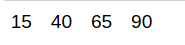If we want to apply the function on all the elements, we just write the apply function like this:

```all_sqrt <- apply(data, 1:2, sqrt)
all_sqrt```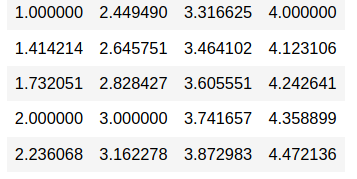What if we want to apply a user-defined function to the data? For example, I have a function that finds the square root of(x – 1) for each row:

```fn = function(x)
{
return(sqrt(x - 1))
}```

We then apply this function along each row:

`apply(data, 1, fn)`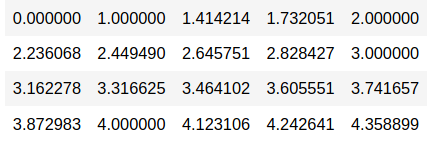So far, we have used functions that take only 1 parameter and applied them to the data. The best part about the apply family is that they work with functions having multiple arguments as well! Let us apply a user-defined function that takes 3 arguments:

```fn = function(x1, x2, x3)
{
return(x1^2 + x2 * x1 + x3)
}```

We take x1 as each value from ‘data’ and x2, x3 as other arguments that will be first declared and then passed through the apply function:

```b = 2
c = 1

# apply along each row:
row_fn <- apply(data, 1, fn, x2 = b, x3 = c)

# apply along each column:
col_fn <- apply(data, 2, fn, x2 = b, x3 = c)```

Let us check row_fn and col_fn

`row_fn`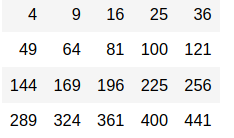`col_fn`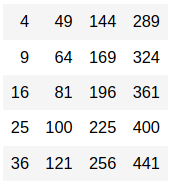The rest of the apply() family follows a similar structure with similar arguments except for a few changes. Let us next use the lapply() function.

## lapply()

The apply() function above has a constraint the data needs to be a matrix of at least 2 dimensions for the apply() function to be performed on it. The lapply() function removes this constraint. Short for list-apply, you can use the lapply function on a list or a vector. Be it a list of vectors or just a simple vector, the lapply() can be used on both. Since we are now dealing with vectors/lists, the lapply function does not need the MARGIN parameter either. That being said, the return type of lapply is also a list.

It takes only the data and the function as the basic parameters:

lapply(X, FUN)

Let us look at some examples:

```# define a list

# use lapply to convert all to lower case
cart_lower <- lapply(cart, tolower)

#output
cart_lower```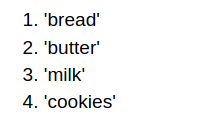We will now take a more complex list of lists:

```data <- list(l1 = c(1, 2, 3, 4),
l2 = c(5, 6, 7, 8),
l3 = c(9, 10, 11, 12))

# apply the 'sum' function on data:
sum_list <- lapply(data, sum)

#output
sum_list```

## sapply()

The sapply() function(short for simplified-apply) is similar to the lapply function. The only difference is the return type of the output – sapply() simplifies the output based on the values returned. I have created a simple table that tells us what type is returned:

Return Value Length of each element Output
List 1 Vector
List > 1 and of the same length Matrix
List > 1 and of the varying length List

We will see examples of all the above scenarios:

#### Scenario 1: Length of each element = 1

```data <- list(l1 = c(1, 2, 3, 4))

# apply the 'sum' function on data:
sum_sapply1 <- sapply(data, sum)

#output
sum_sapply1```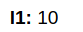Using lapply to see the difference in outputs:

```sum_lapply1 <- lapply(data, sum)
sum_lapply1```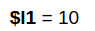#### Scenario 2: Length of each element > 1 and same

```data <- list(l1 = c(1, 2, 3, 4),
l2 = c(5, 6, 7, 8),
l3 = c(9, 10, 11, 12))

# apply the 'sum' function on data:
sum_sapply2 <- sapply(data, sum)

#output
sum_sapply2```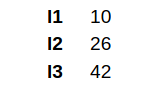What output does lapply() give us?

```sum_lapply2 <- lapply(data, sum)
sum_lapply2```

#### Scenario 3: Length of each element > 1 and different

```data <- list(l1 = c(1, 2, 3),
l2 = c(5, 6, 7, 8),
l3 = c(9, 10))

# apply the 'sum' function on data:
sum_sapply3 <- sapply(data, sum)

#output
sum_sapply3```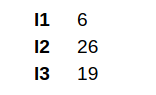Let us compare it with the output of lapply() on the same data:

```sum_lapply3 <- lapply(data, sum)

#output
sum_lapply3```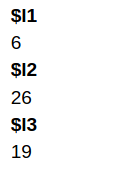You can see how the output differs from lapply above which returned a list

## vapply()

Coming to the vapply() function. The trio of lapply(), apply(), and vapply() are specially tailored for vectors of all types. Unlike lapply() and sapply() which decide the data type of the output for us, vapply() allows us to choose the data type of the output structure. Thus, the parameters for vapply() are:

vapply(X, FUN, FUN.VALUE)

Here FUN.VALUE is used to provide the data type you want.

This is most useful when our lost/vectors contain a mix of numbers and strings:

```data <- list(l1 = c(1, 2, 3, 4),
l2 = c(5, 6, 7, 8),
l3 = c(9, 10, 11, 12),
l4 = c("a", "b", "c", "a"))

# apply the 'max' function on data:
sum_vapply <- vapply(data, max, numeric(1))```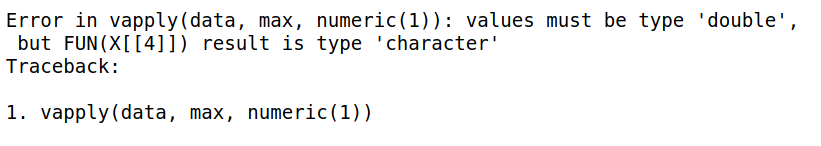As expected, we got an error because it is not possible to compute the max value from a list of characters. The numeric(1) specifies that we want the output to be individual numeric values where the length of each element is 1. What if we use lapply() or sapply()?

```lapply(data, max)
sapply(data, max)```

Thus, we can see that both lapply() and sapply() actually provided outputs for the same. In fact, sapply() even converted the output to a vector of type character. Ideally, this is not what we want. Generally, this is how we use the vapply() function

```data <- list(l1 = c(1, 2, 3, 4),
l2 = c(5, 6, 7, 8),
l3 = c(9, 10, 11, 12))

max_vapply <- vapply(data, max, numeric(1))

max_vapply```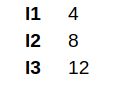Thus, it is always better to use vapply() when you are working with data frames that have different datatypes of features.

## tapply()

In the simplest terms, tapply() allows us to divide the data into groups and perform the operation on each group. Thus, when you provide a vector as input, tapply() performs the specified operation on each subset of the vector. The parameters it takes are:

tapply(X, INDEX, FUN)

where INDEX represents the factor you want to use to separate the data. Sounds familiar? Yes, tapply() is nothing but a simple way to perform a groupy operation and apply some function on this grouped data!

To observe how tapply() works let us create 2 simple vectors

```item_cat <- c("HOME", "SNACKS", "BEVERAGE", "STORAGE", "CLEANING", "STORAGE", "HOME", "BEVERAGE", "ELECTRONICS", "SNACKS")
item_qty <-c(25, 30, 45, 66, 15, 50, 35, 20, 15, 35)```

Let us now use tapply to get the mean quantity of each item category:

`tapply(item_qty, item_cat, mean)`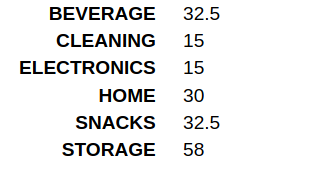What did the tapply() function do? We grouped the item_qty vector by the item_cat vector to create subsets of the vectors. We then calculate the mean of each subset.

What makes it so easy to use tapply() is that it automatically takes the unique values from the item_cat vector and applies the function we want on the data almost instantly. We can even get more than one value on each subset:

`tapply(item_qty, item_cat, function(x) c(mean(x), sum(x)))`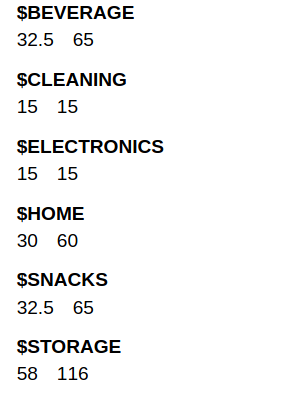Now, we come to the last function in the apply() family of functions – the mapply() function.

## mapply()

mapply() stands for multivariate-apply and basically is a multivariate version of sapply(). The mapply function is best explained by examples – so let us use it first and then try to understand how it works.

Let us first take a function that generally does not take 2 lists or 2 vectors as arguments – for example, the max function. We take 2 lists first:
```list1 <- list(a = c(1, 2, 3), b = c(4, 5, 6), c = c(7, 8, 9))
list2 <- list(a = c(10, 11, 12), b = c(13, 14, 15), c = c(16, 17, 18))```

Now, what if we want to find the max values between each pair of lists elements?

`max(list1\$a, list2\$a)`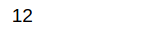Now, this function cannot be applied at the same time across all elements of list1 and list2. In such cases, we use the mapply() function:

`mapply(function(num1, num2) max(c(num1, num2)), list1, list2)`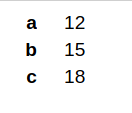Thus, the mapply function is used to perform functions on data that don’t generally accept multiple lists/vectors as arguments. It is also useful when you want to create new columns. Let us first create a dataframe from the matrix we defined initially:

`df <- as.data.frame(data)`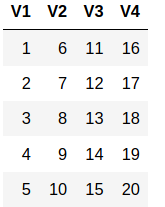We will now create a new variable that contains the product of columns V1 and V3:

`mapply(function(x, y) x/y, df\$V1, df\$V3)`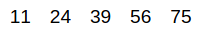Thus, we see that mapply is a really handy function when working with data frames.

Now, let us see how to use these functions on real-life datasets. For simplicity, let us take up the iris dataset:

```iris_df<-datasets::iris

##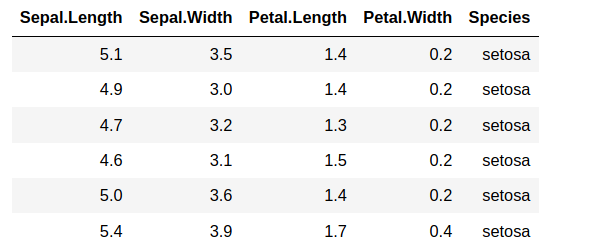We can now compute the mean of sepal length and sepal width of each row using the apply() function:

`iris_df['Sepal_mean'] <- apply(iris_df[c("Sepal.Length", "Sepal.Width")], 1, mean)`

##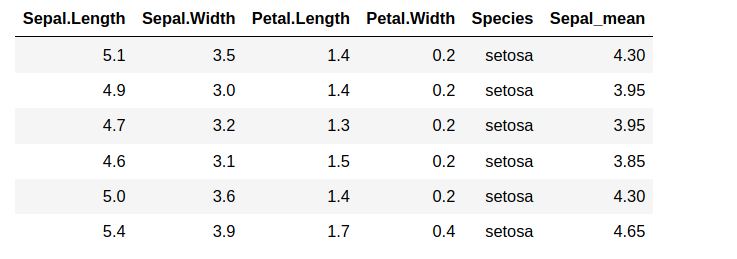Similarly, we can obtain summary values of each column for each species in the dataframe:

`tapply(iris_df\$Sepal.Width, iris_df\$Species, mean)`

##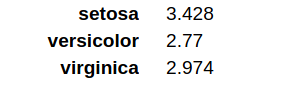We can also create a new column showing the sum of petal length and petal width using the mapply() function:

`iris_df['Sum_Petal'] <- mapply(function(x, y) x+y, iris_df\$Petal.Length, iris_df\$Petal.Width)`

## End Notes

So far, we learned the various functions in the apply() family of functions in R. These set of functions provide extremely efficient means to apply various operations on data in a split second. The article covers the basics of these functions for the purpose of making you understand how these functions work.

I encourage you to try more complicated functions on more complicated datasets to fully understand how useful these functions are. How have these functions helped you in working with datasets in R? Please share your replies and any questions below!